# Describe the various components used for message integrity in cryptography ?

The types of functions that may be used to produce an authenticator may be grouped into three classes

• Hash function
• A function that maps a message of any length into a fixed-length hash value which serves as the authenticator.
• Message encryption
• The ciphertext of the entire message serves as its authenticator.
• Message authentication code (MAC)
• A function of the message and a secret key that produces a fixed-length value that serves as the authenticator
• Message authentication mechanism verify the integrity of a message –  it assures that data received are exactly as sent (i.e., contain no modification, insertion, deletion, or replay).
• It also checks that the identity of the sender is valid.
• When a hash function is used to provide message authentication, the hash function value is often referred to as a message digest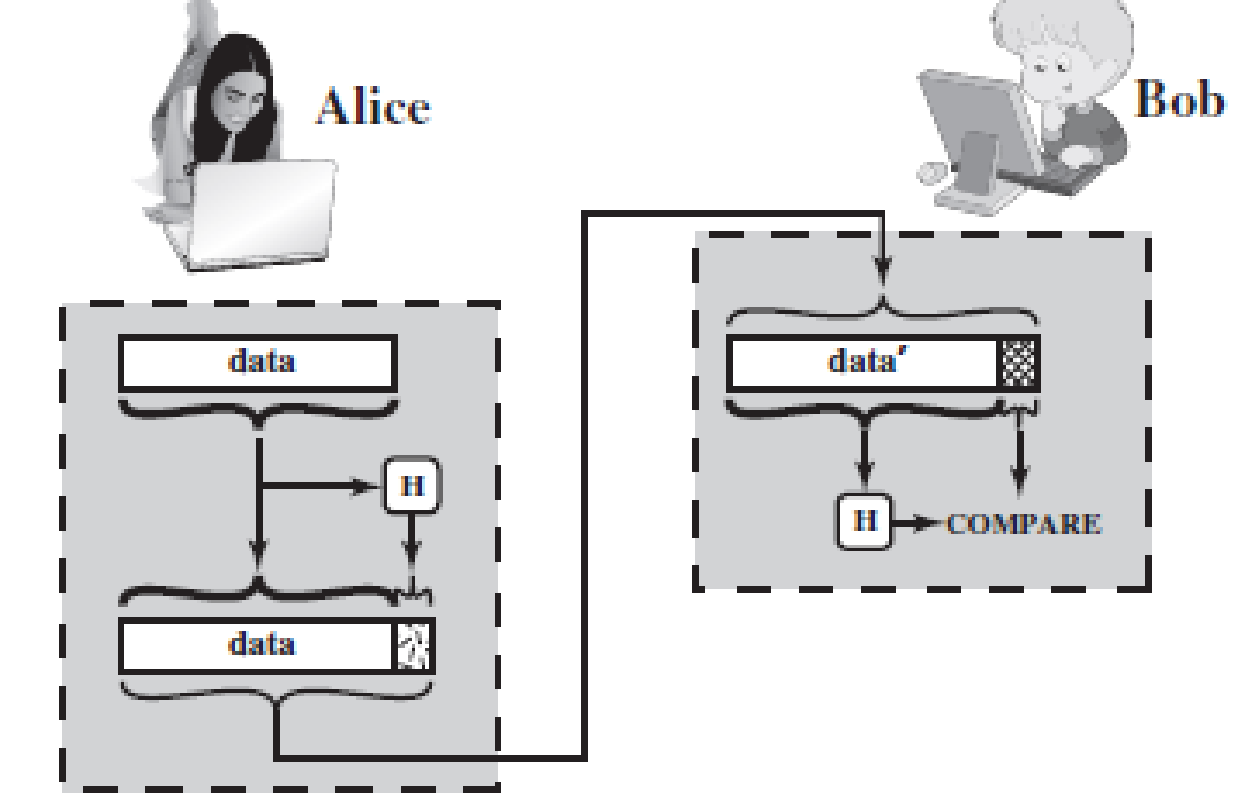• The sender computes a hash value as a function of the bits in the message and transmits both the hash value and the message.
• The receiver performs the same hash calculation on the message bits and compares this value with the incoming hash value.
• If there is a mismatch, the receiver knows that the message (or possibly the hash value) has been altered

Use of a Hash Function for Message Authentication  cntd..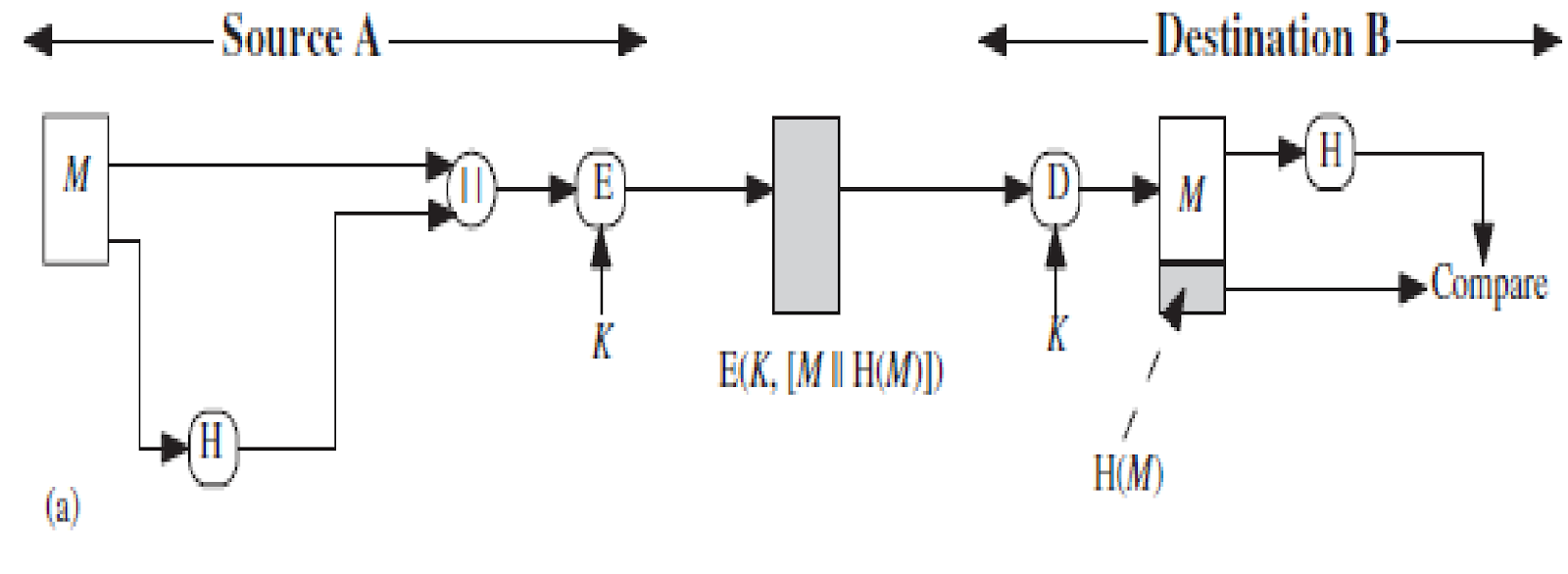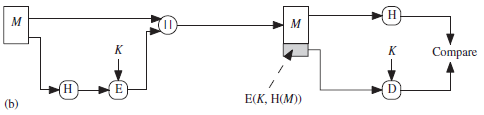1. The message plus concatenated hash code is encrypted using symmetric encryption. Because only A and B share the secret key, the message must have come from A and has not been altered.
• Because encryption is applied to the entire message plus hash code, confidentiality is also provided.
1. Only the hash code is encrypted, using symmetric encryption.
• This reduces the processing burden for those applications that do not require confidentiality.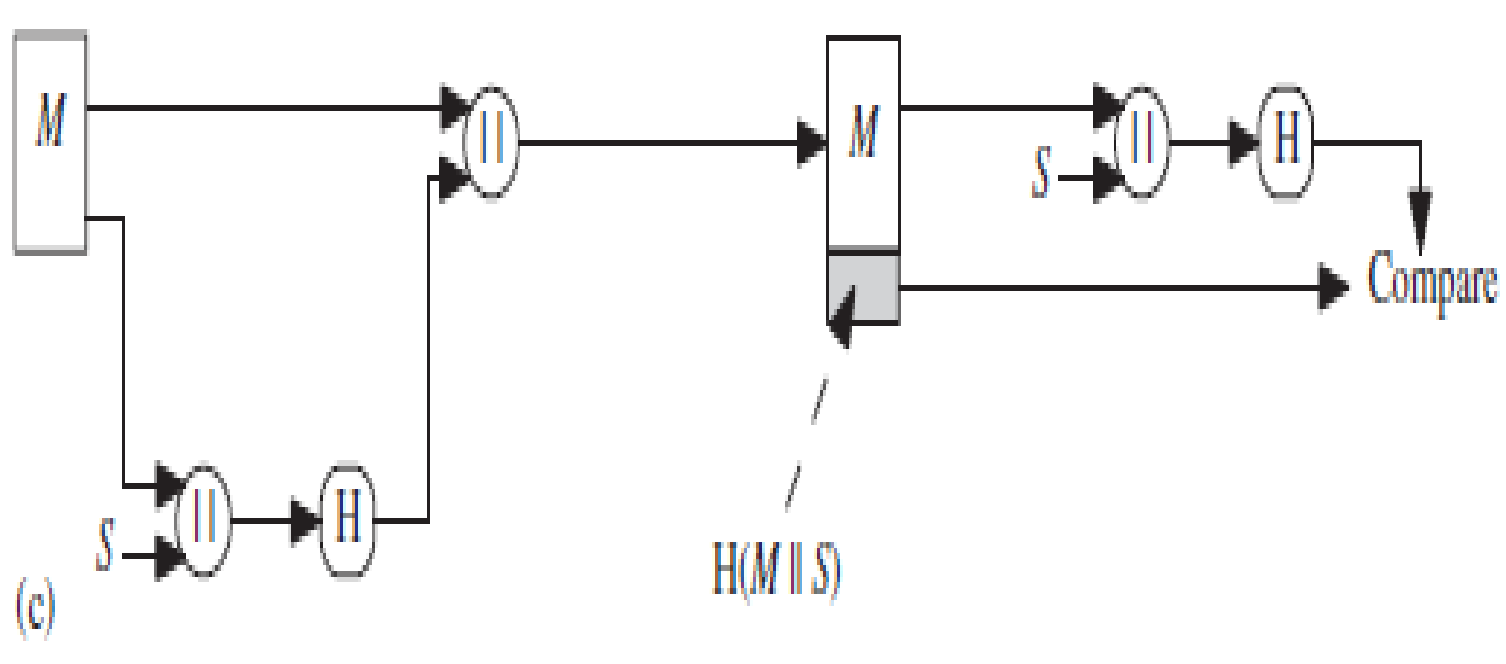1. It is possible to use a hash function but no encryption for message authentication. The technique assumes that the two communicating parties share a common secret value S.
• A computes the hash value over the concatenation of M and S and appends the resulting hash value to M.  Because B possesses S, it can recomputed the hash value to verify.
• Because the secret value itself is not sent, an opponent cannot modify an intercepted message and cannot generate a false message.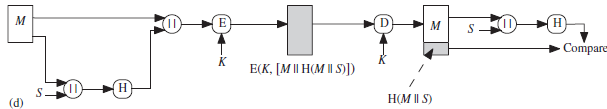Confidentiality can be added to the approach of method (c) by  encrypting the entire message plus the hash code.

Basic Uses of Message Encryption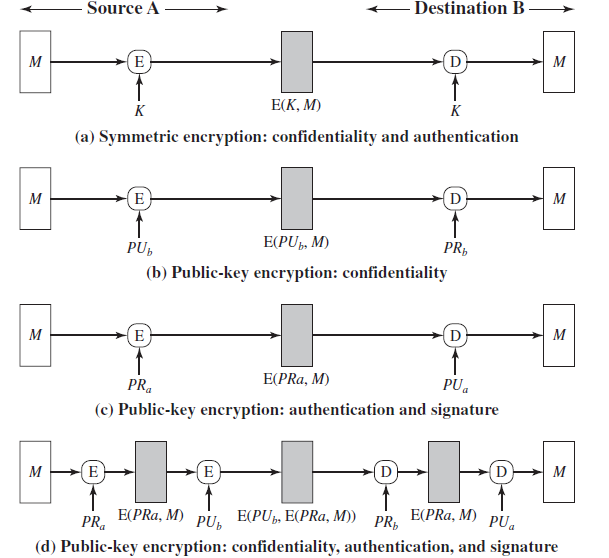Message Authentication Code (MAC)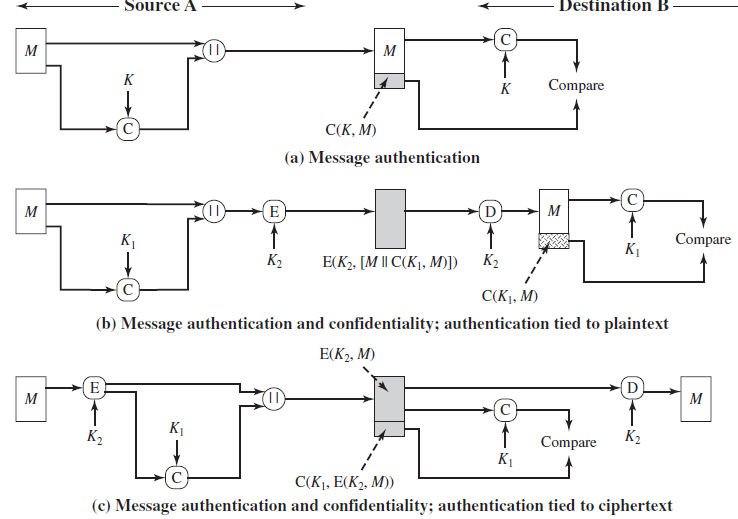• A secret key K is used to generate a small fixed-size block of data, known as a cryptographic checksum  or MAC, that is appended to the message.

MAC =  C(K , M )   where

C = MAC function

K = shared secret key

M = input message

An alternative authentication technique involves the use of a secret key to generate

a small fixed-size block of data, known as a cryptographic checksum  or MAC, that is

appended to the message. This technique assumes that two communicating parties,

say A and B, share a common secret key K. When A has a message to send to B, it

calculates the MAC as a function of the message and the key:

MAC =  C(K , M )

where

M =  input message

C =  MAC function

K =  shared secret key

MAC =  message authentication code

The message plus MAC are transmitted to the intended recipient. The recipient

performs the same calculation on the received message, using the same secret key,

to generate a new MAC. The received MAC is compared to the calculated MAC

(Figure 12.4a). If we assume that only the receiver and the sender know the identity

of the secret key, and if the received MAC matches the calculated MAC, then

1.  The receiver is assured that the message has not been altered. If an attacker

alters the message but does not alter the MAC, then the receiver’s calculation

of the MAC will differ from the received MAC. Because the attacker is assumed

not to know the secret key, the attacker cannot alter the MAC to correspond

to the alterations in the message.

2.  The receiver is assured that the message is from the alleged sender. Because

no one else knows the secret key, no one else could prepare a message with a

proper MAC.

3. If the message includes a sequence number (such as is used with HDLC, X.25,

and TCP), then the receiver can be assured of the proper sequence because an

attacker cannot successfully alter the sequence number.

A MAC function is similar to encryption. One difference is that the MAC

algorithm need not be reversible, as it must be for decryption. In general, the MAC

function is a many-to-one function. The domain of the function consists of messages

of some arbitrary length, whereas the range consists of all possible MACs and all

possible keys. If an n -bit MAC is used, then there are 2n  possible MACs, whereas

there are N  possible messages with N >>  2n . Furthermore, with a k -bit key, there

are 2k  possible keys.

The process depicted in Figure 12.4a provides authentication but not confidentiality,

because the message as a whole is transmitted in the clear. Confidentiality

can be provided by performing message encryption either after (Figure 12.4b) or

before (Figure 12.4c) the MAC algorithm. In both these cases, two separate keys are

needed, each of which is shared by the sender and the receiver. In the first case, the

MAC is calculated with the message as input and is then concatenated to the message.

The entire block is then encrypted. In the second case, the message is encrypted

first. Then the MAC is calculated using the resulting ciphertext and is concatenated

to the ciphertext to form the transmitted block. Typically, it is preferable to tie the

authentication directly to the plaintext, so the method of Figure 12.4b is used.

It is assumed that only the receiver and the sender know the identity of the secret key, and if the received MAC matches the calculated MAC, then

1. The receiver is assured that the message has not been altered.
• If an attacker alters the message but does not alter the MAC, then the receiver’s calculation of the MAC will differ from the received MAC. Because the attacker is assumed not to know the secret key, the attacker cannot alter the MAC to correspond to the alterations in the message.
2.  The receiver is assured that the message is from the alleged sender.
• Because no one else knows the secret key, no one else could prepare a message with a proper MAC.
3. If the message includes a sequence number (such as is used with HDLC, and TCP), then the receiver can be assured of the proper sequence because an attacker cannot successfully alter the sequence number.

In assessing the security of a MAC function, we need to consider the types of attacks that may be mounted against it. Hence it needs to satisfy the listed requirements.

The first requirement deals with message replacement attacks, in which an opponent is able to construct a new message to match a given MAC, even though the opponent does not know and does not learn the key.

The second requirement deals with the need to thwart a brute-force attack based on chosen plaintext.

The final requirement dictates that the authentication algorithm should not be weaker with respect to certain parts or bits of the message than others.

• A MAC function is similar to encryption. One difference is that the MAC algorithm need not be reversible, as it must be for decryption.
• In general, the MAC function is a many-to-one function. The domain of the function consists of messages of some arbitrary length, whereas the range consists of all possible MACs and all possible keys.
•  If an n -bit MAC is used, then there are 2n  possible MACs, whereas there are N  possible messages with N >>  2n . Furthermore, with a k -bit key, there are 2k  possible keys.
• Typically, it is preferable to tie the authentication directly to the plaintext, so the method of Figure b is used.

Implementation of MAC algorithms

• In essence, the security of MAC depends on the security of the underlying algorithm.
• There is much more variety in the structure of MACs than in hash functions, so it is difficult to generalize about the cryptanalysis of MACs.

We will consider two types of MACs developed:

1. MAC Based on Hash Functions: HMAC
2. Cipher based MAC: DAA and CMAC